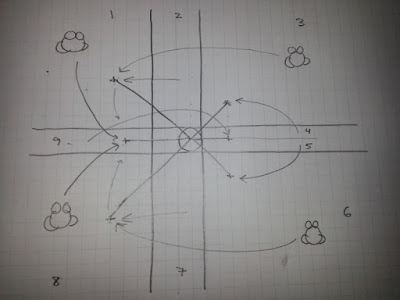## Wednesday, July 29, 2015

### Making a Smarter Opponent

The frog soccer game made with Phaser is coming along nicely.

After getting the ball-out-of-bounds logic working pretty well over the weekend I was thinking more about the opponent logic. The pattern followed by the other frog seemed to work but was too simple. I wanted something a little better.

The frog should pick a different target location based on her position relative to the ball, but there are a lot of zones to consider. Is she directly in line with the ball on the X or Y axis? Is she below the ball on the Y axis? Is she on the goal side of the ball? What should be done to get her to the next location?

When dealing with these kinds of problems I find it helpful to draw a picture. I keep a graph paper tablet around for times such as these.Frog zone sketch
I sketched out the different zones the frog could be in relative to the ball and what I believed the target should be when in each of those zones. After that, it was easy to translate these behaviors into code.

```function setTarget(o, b) {

var zone = 0;

if (o.position.x < (b.position.x - 90) && o.position.y < (b.position.y - 5)) {
zone = 1;
} else if (o.position.x >= (b.position.x - 90) && o.position.x <= (b.position.x + 30) && o.position.y < (b.position.y - 30)) {
zone = 2;
} else if (o.position.x > (b.position.x + 30) && o.position.y < (b.position.y - 30)) {
zone = 3;
} else if (o.position.x > (b.position.x + 30) && o.position.y <= (b.position.y) && o.position.y > (b.position.y - 30)) {
zone = 4;
} else if (o.position.x > (b.position.x + 30) && o.position.y > (b.position.y) && o.position.y < (b.position.y + 30)) {
zone = 5;
} else if (o.position.x > (b.position.x + 30) && o.position.y > (b.position.y + 30)) {
zone = 6;
} else if (o.position.x >= (b.position.x - 90) && o.position.x <= (b.position.x + 30) && o.position.y > (b.position.y + 30)) {
zone = 7;
} else if (o.position.x < (b.position.x - 90) && o.position.y > (b.position.y + 5)) {
zone = 8;
} else if (o.position.x < (b.position.x - 90) && o.position.y >= (b.position.y - 5) && o.position.y <= (b.position.y + 5)) {
zone = 9;
}

var ret = {
x: o.position.x,
y: o.position.y
};

if (zone === 1) {
ret.x = b.position.x - 180;
ret.y = b.position.y + 20;
} else if (zone === 2) {
ret.x = b.position.x - 180;
ret.y = b.position.y - 150;
} else if (zone === 3) {
ret.x = b.position.x - 180;
ret.y = b.position.y - 150;
} else if (zone === 4) {
ret.x = b.position.x + 90;
ret.y = b.position.y - 90;
} else if (zone === 5) {
ret.x = b.position.x + 90;
ret.y = b.position.y + 90;
} else if (zone === 6) {
ret.x = b.position.x - 180;
ret.y = b.position.y + 150;
} else if (zone === 7) {
ret.x = b.position.x - 180;
ret.y = b.position.y + 150;
} else if (zone === 8) {
ret.x = b.position.x - 180;
ret.y = b.position.y - 20;
} else if (zone === 9) {
ret.x = b.position.x + 90;
ret.y = b.position.y - 15;
}

return ret;

}
```

Making the sketch plan in advance made it much easier to code, and it worked well on the first try. It still needed some fine-tuning, but it was really close. I know I could have combined some things in the code above but I wanted to keep the logic very clear and clean so I could tune it. If necessary I can always optimize it later. I tend to favor readability over other factors.

I made another video of the frogs playing. The computer-controlled frog starts out on the right side of the ball but moves herself around to the left and starts moving toward the goal. She chases it quite well when I manage to kick it away from her.

Remember, the full source code is on GitHub if you want to try improving the logic.

And that's all I have for today. Like I said, I only work on this for a few minutes each day...and this might be the last update this week. I have something different going on for Friday's comic. But I'm getting ahead of myself. Here's today's comic:Amphibian.com comic for 29 July 2015